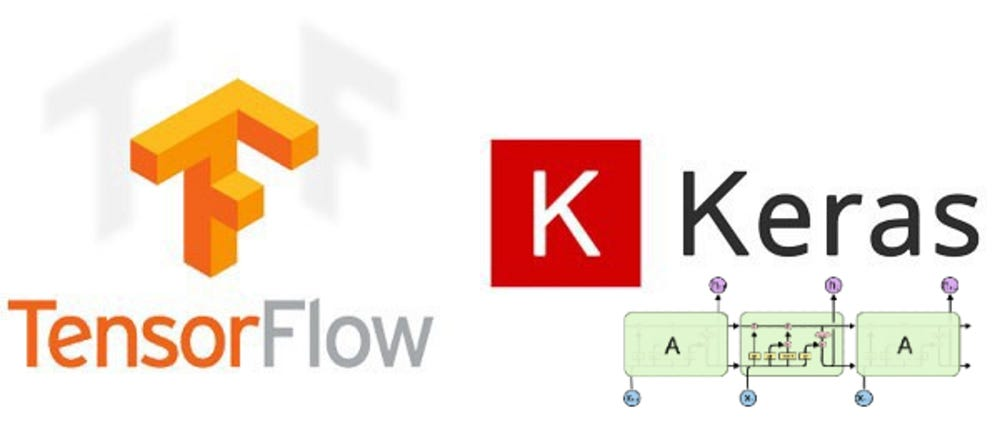# Deep Learning LSTM for Sentiment Analysis in Tensorflow with Keras API.

Source: Deep Learning on Medium• calling text_to_sequence replaces the words in a sentence with their respective associated numbers. This transforms each sentence into sequences of numbers.
`print(tweet)print(encoded_docs)`

From the above result, you can see the tweet is encoded as a sequence of numbers. eg. to and the are converted to 1 and 2 respectively.
Check the word index above to verify.

The sentences or tweets have different number of words, therefore, the length of the sequence of numbers will be different.
Our model requires inputs to have equal lengths, so we will have to pad the sequence to have the chosen length of inputs. This is done by calling the pad_sequence method with a length of 200.
All input sequences will have a length of 200.

`print(padded_sequence)`

# Build Model

Now that we have the inputs processed. It’s time to build the model.

`# Build the modelfrom tensorflow.keras.models import Sequentialfrom tensorflow.keras.layers import LSTM,Dense, Dropout,from tensorflow.keras.layers import SpatialDropout1Dfrom tensorflow.keras.layers import Embeddingembedding_vector_length = 32model = Sequential()model.add(Embedding(vocab_size, embedding_vector_length,  input_length=200) )model.add(SpatialDropout1D(0.25))model.add(LSTM(50, dropout=0.5, recurrent_dropout=0.5))model.add(Dropout(0.2))model.add(Dense(1, activation='sigmoid'))model.compile(loss='binary_crossentropy',optimizer='adam',  metrics=['accuracy'])print(model.summary())`

This is where we get to use the LSTM layer. The model consists of an embedding layer, LSTM layer and a Dense layer which is a fully connected neural network with sigmoid as the activation function.

Dropouts are added in-between layers and also on the LSTM layer to avoid overfitting.

# LSTM

Long Short Term Memory networks — usually just called “LSTMs” — are a special kind of RNN, capable of learning long-term dependencies. They were introduced by Hochreiter & Schmidhuber (1997), and were refined and popularized by many people in following work.1 They work tremendously well on a large variety of problems, and are now widely used.

LSTMs are explicitly designed to avoid the long-term dependency problem. Remembering information for long periods of time is practically their default behavior, not something they struggle to learn!

# Train Model

`history = model.fit(padded_sequence,sentiment_label, validation_split=0.2, epochs=5, batch_size=32)`